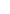## Semiconductors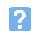1. Examples of semiconductor materials are: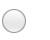a) aluminium and steelb) brass and zincc) quartz and silicad) silicon and germanium.2. The component shown below is: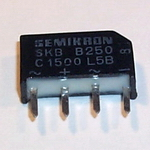a) a signal diodeb) a bridge rectifierc) a zener diode regulatord) a miniature transformer.3. A perfect diode would have:a) zero forward resistance and zero reverse resistanceb) zero reverse resistance and infinite forward resistancec) zero forward resistance and infinite reverse resistanced) infinite forward resistance and infinite reverse resistance.4. When a bipolar junction transistor is used as a conventional amplifer:a) both the base-emitter and collector-base junctions are forward biasedb) both the base-emitter and collector-base junctions are reverse biasedc) the base-emitter is forward biased whilst the collector-base is reverse biasedd) the base-emitter is reverse biased whilst the collector-base is forward biased.5. The forward voltage drop for germanium diode is approximately:a) 0.2 Vb) 0.5 Vc) 0.6 Vd) 0.8 V.6. The typical operating forward current for a standard LED is:a) 15 mAb) 150 mAc) 300 mAd) 1 A.7. The connections to a silicon controlled rectifier are labelled:a) anode, cathode, baseb) anode, cathode, gatec) anode, cathode, emitterd) anode, cathode, trigger.8. In the diagram of the rectifier shown below, which connection is the positive output terminal?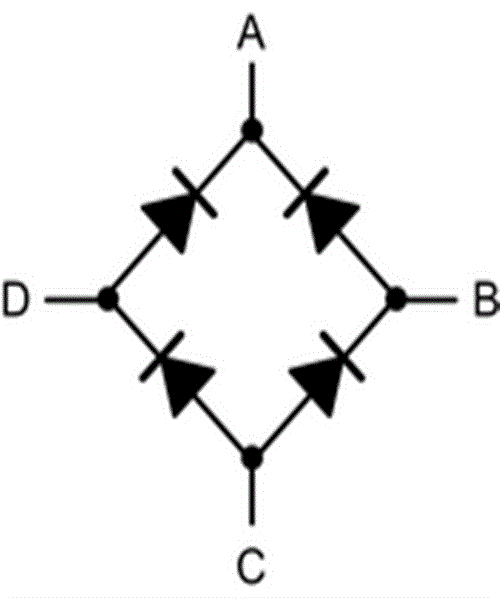a) Ab) Bc) Cd) D.9. An application for a zener diode is:a) a rectifierb) a current limiterc) a power indicatord) a voltage regulator.10. The component shown below is: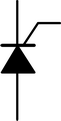a) a zener diodeb) a signal diodec) a variable capacitance dioded) a silicon controlled rectifier.11. The component shown below is: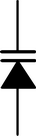a) a zener diodeb) a signal diodec) a variable capacitance dioded) a silicon controlled rectifier.12. The connection marked X in the diagram below is: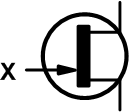a) baseb) emitterc) gated) source.
This is more feedback!
This is the feedback!